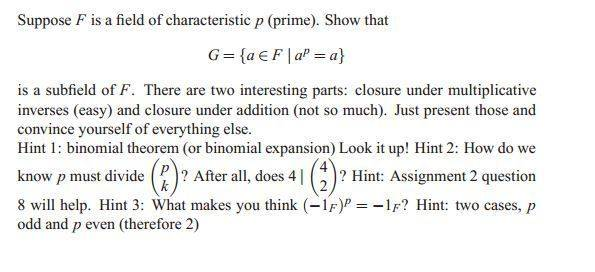# Subfield proof

stripes

## Homework Statement## The Attempt at a Solution

Well thankfully I just have to present closure under mult. inverses and closure under addition. But I seem to be going in circles...if a is in G, then we need to show that a-1 is also in G.

So a*a-1 = 1F, but is a-1 in G...so we can write a = ap, then if we can write a-1 = (ap)-1, then we'll be good. But how the heck do I do that?

For closure under addition, I'm not really sure how to use the binomial theorem here, since we know that a + b means we can write them both as ap + bp, and now we've got to show that ap + bp can be written as (α + β)p. Not sure how to get this one started either.

Any help is appreciated, thanks in advance.

Homework Helper

## Homework Statement## The Attempt at a Solution

Well thankfully I just have to present closure under mult. inverses and closure under addition. But I seem to be going in circles...if a is in G, then we need to show that a-1 is also in G.

So a*a-1 = 1F, but is a-1 in G...so we can write a = ap, then if we can write a-1 = (ap)-1, then we'll be good. But how the heck do I do that?

For closure under addition, I'm not really sure how to use the binomial theorem here, since we know that a + b means we can write them both as ap + bp, and now we've got to show that ap + bp can be written as (α + β)p. Not sure how to get this one started either.

Any help is appreciated, thanks in advance.

I'm not sure why you are stumbling around with the multiplicative proof. If a=a^p then a^(-1)=(a^p)^(-1). But that's the same thing as (a^(-1))^p isn't it? And for addition, you use the binomial theorem to prove that (a+b)^p=a^p+b^p in F. This is where the field having characteristic p is really important.